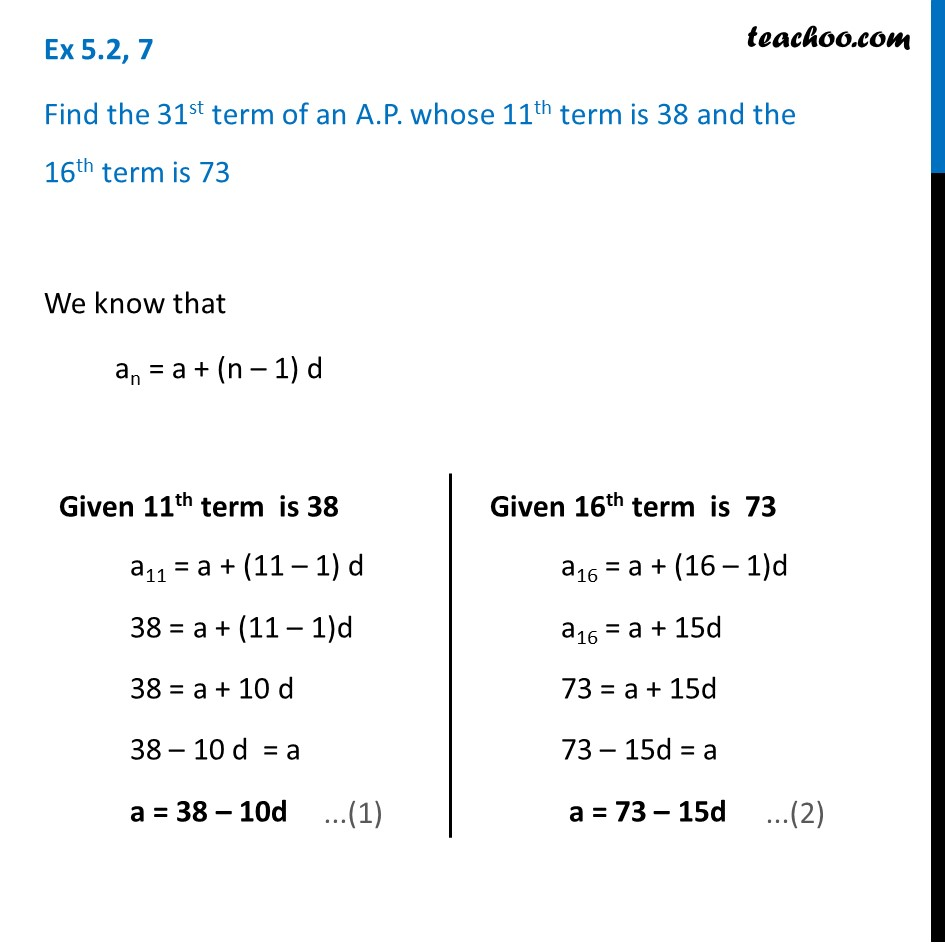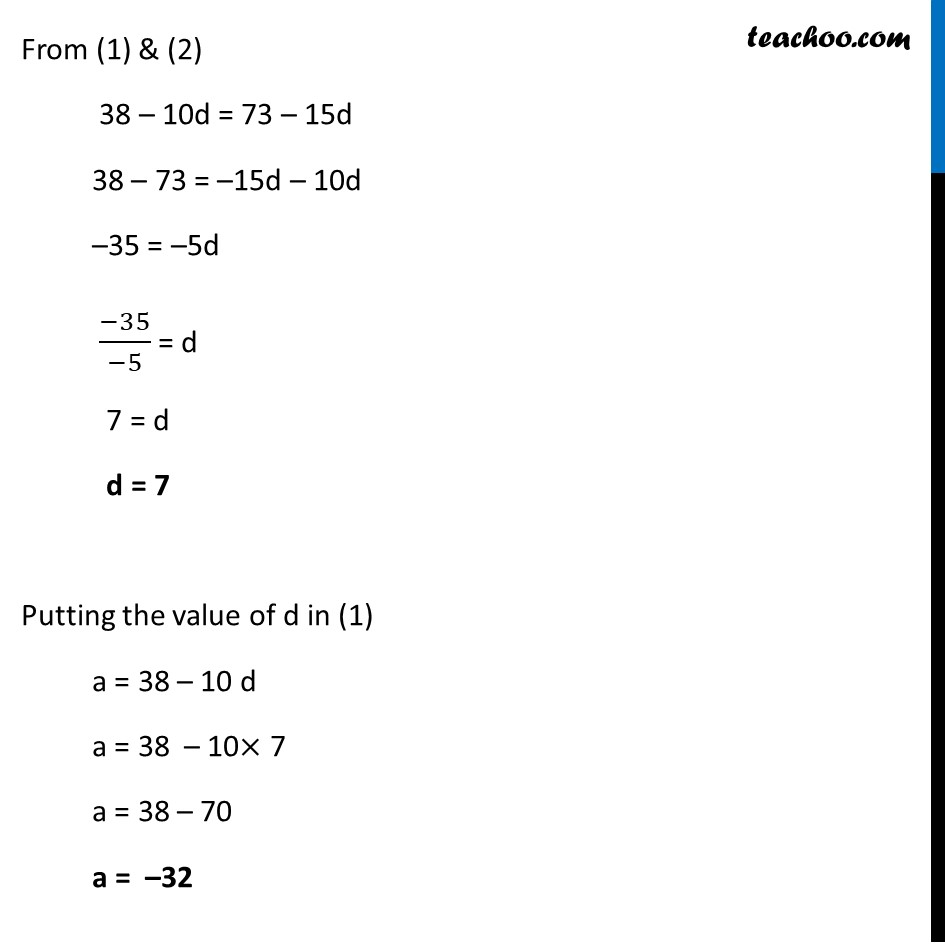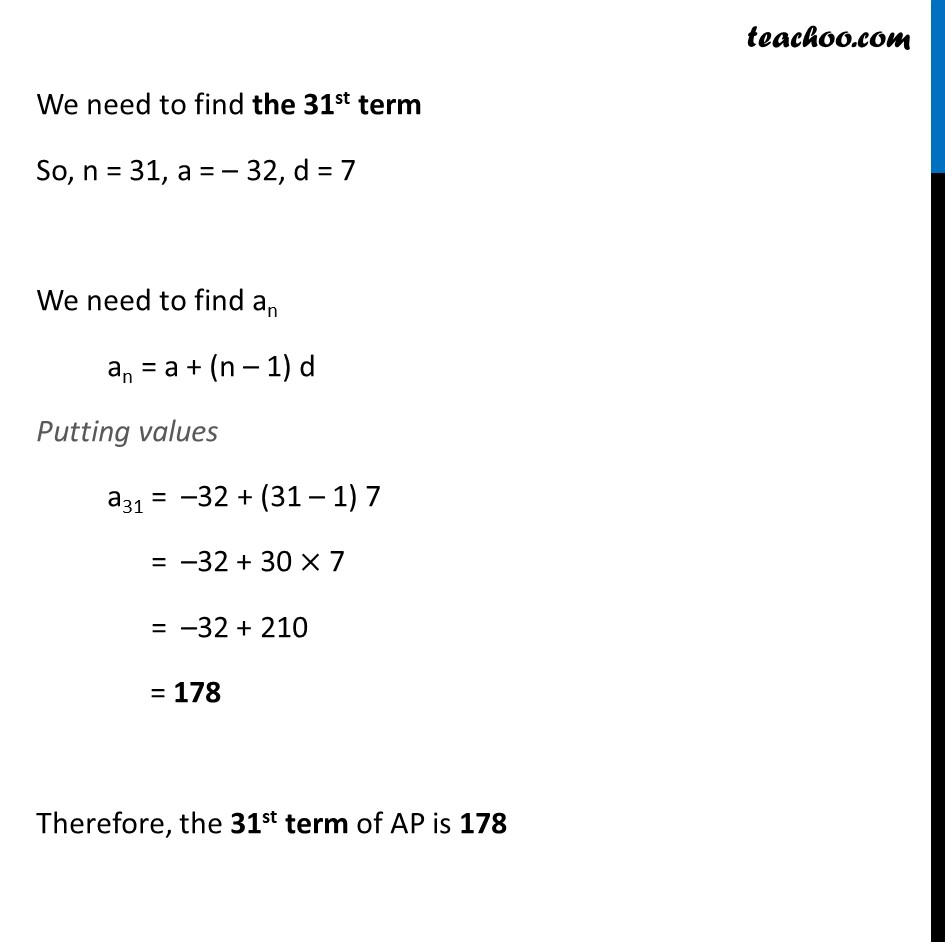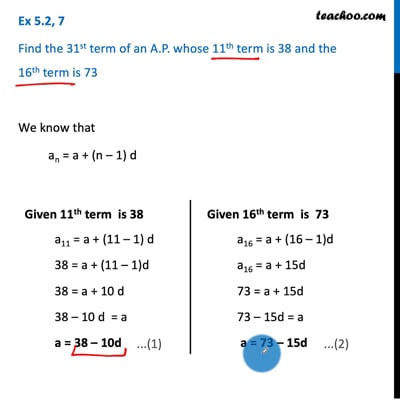Finding nth term

Chapter 5 Class 10 Arithmetic Progressions (Term 2)
Concept wiseThis video is only available for Teachoo black users

### Transcript

Ex 5.2, 7 Find the 31st term of an A.P. whose 11th term is 38 and the 16th term is 73 We know that an = a + (n – 1) d Given 11th term is 38 a11 = a + (11 – 1) d 38 = a + (11 – 1)d 38 = a + 10 d 38 – 10 d = a a = 38 – 10d Given 16th term is 73 a16 = a + (16 – 1)d a16 = a + 15d 73 = a + 15d 73 – 15d = a a = 73 – 15d From (1) & (2) 38 – 10d = 73 – 15d 38 – 73 = –15d – 10d –35 = –5d (−35)/(−5) = d 7 = d d = 7 Putting the value of d in (1) a = 38 – 10 d a = 38 – 10× 7 a = 38 – 70 a = –32 We need to find the 31st term So, n = 31, a = – 32, d = 7 We need to find an an = a + (n – 1) d Putting values a31 = –32 + (31 – 1) 7 = –32 + 30 × 7 = –32 + 210 = 178 Therefore, the 31st term of AP is 178